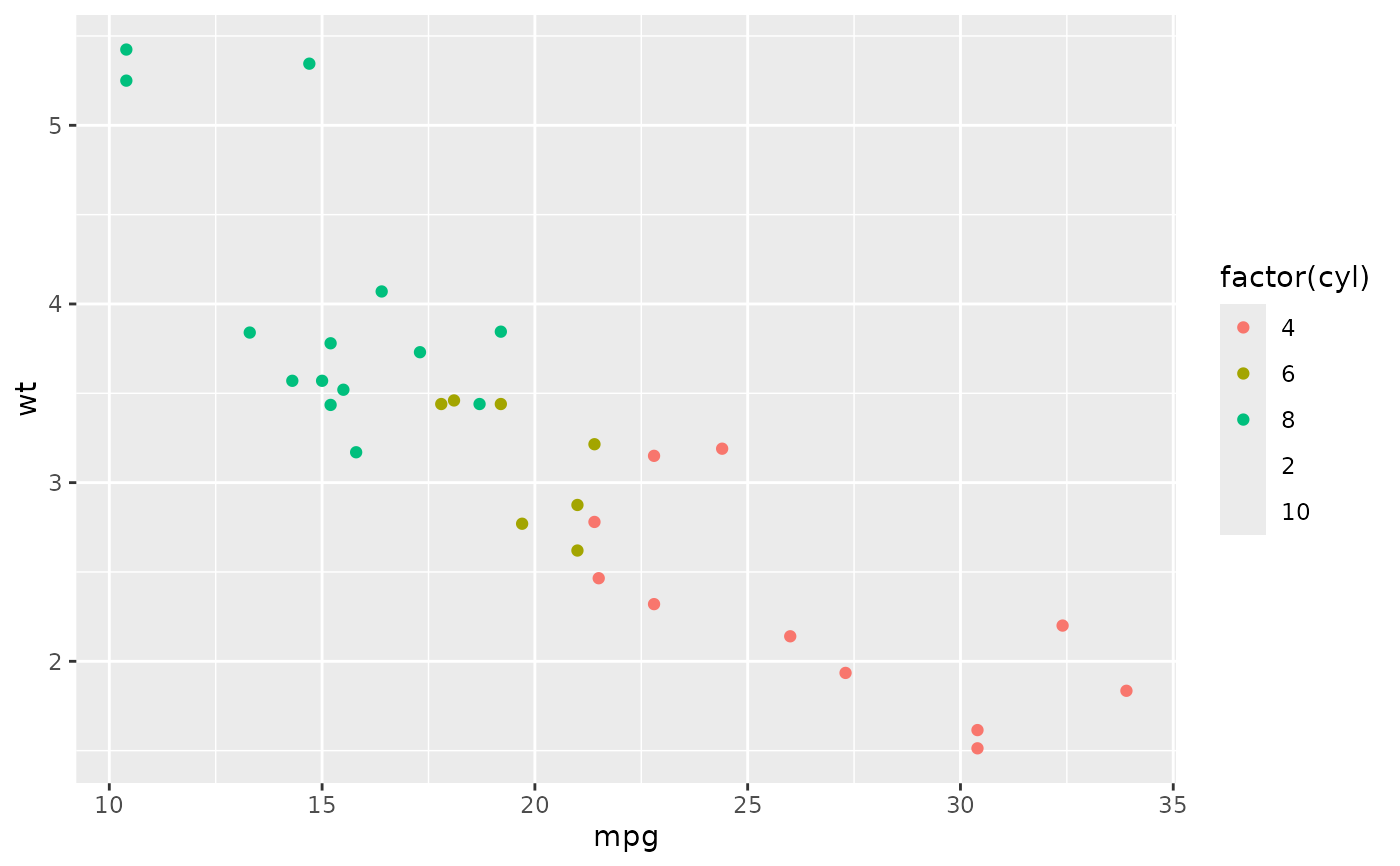Sometimes you may want to ensure limits include a single value, for all panels or all plots. This function is a thin wrapper around geom_blank() that makes it easy to add such values.

## Usage

expand_limits(...)

## Arguments

...

named list of aesthetics specifying the value (or values) that should be included in each scale.

## Examples

p <- ggplot(mtcars, aes(mpg, wt)) + geom_point()
p + expand_limits(x = 0)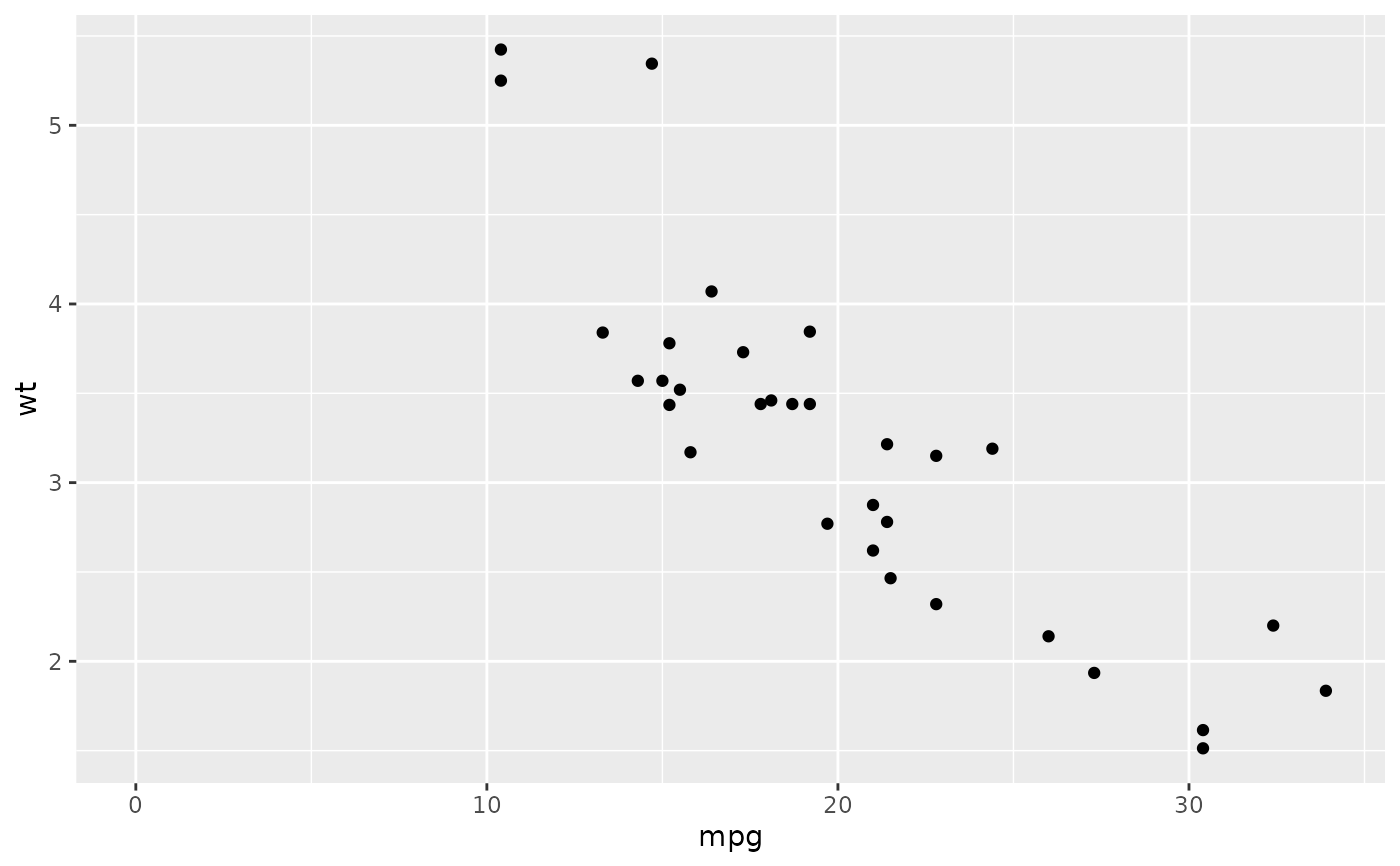p + expand_limits(y = c(1, 9))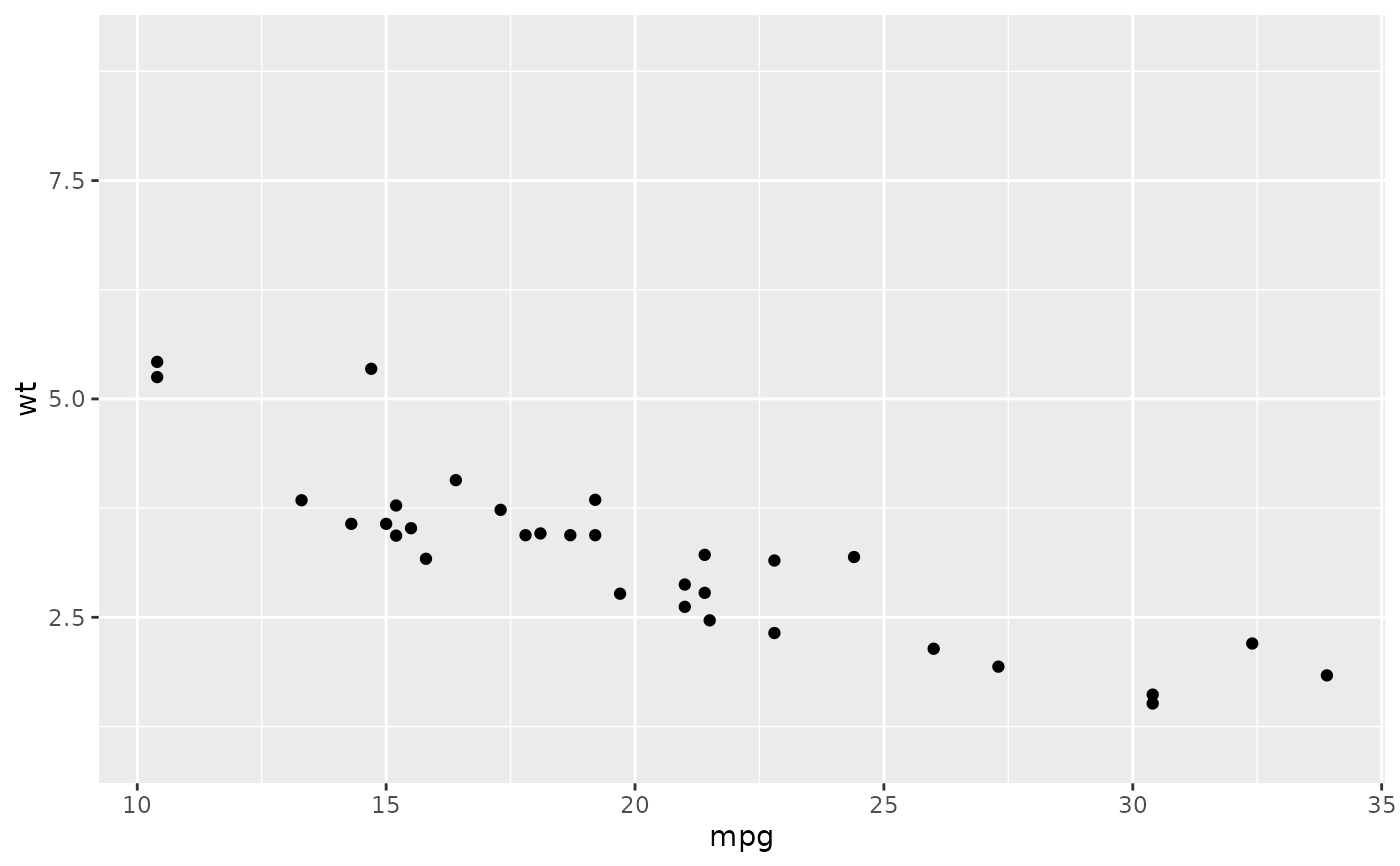p + expand_limits(x = 0, y = 0)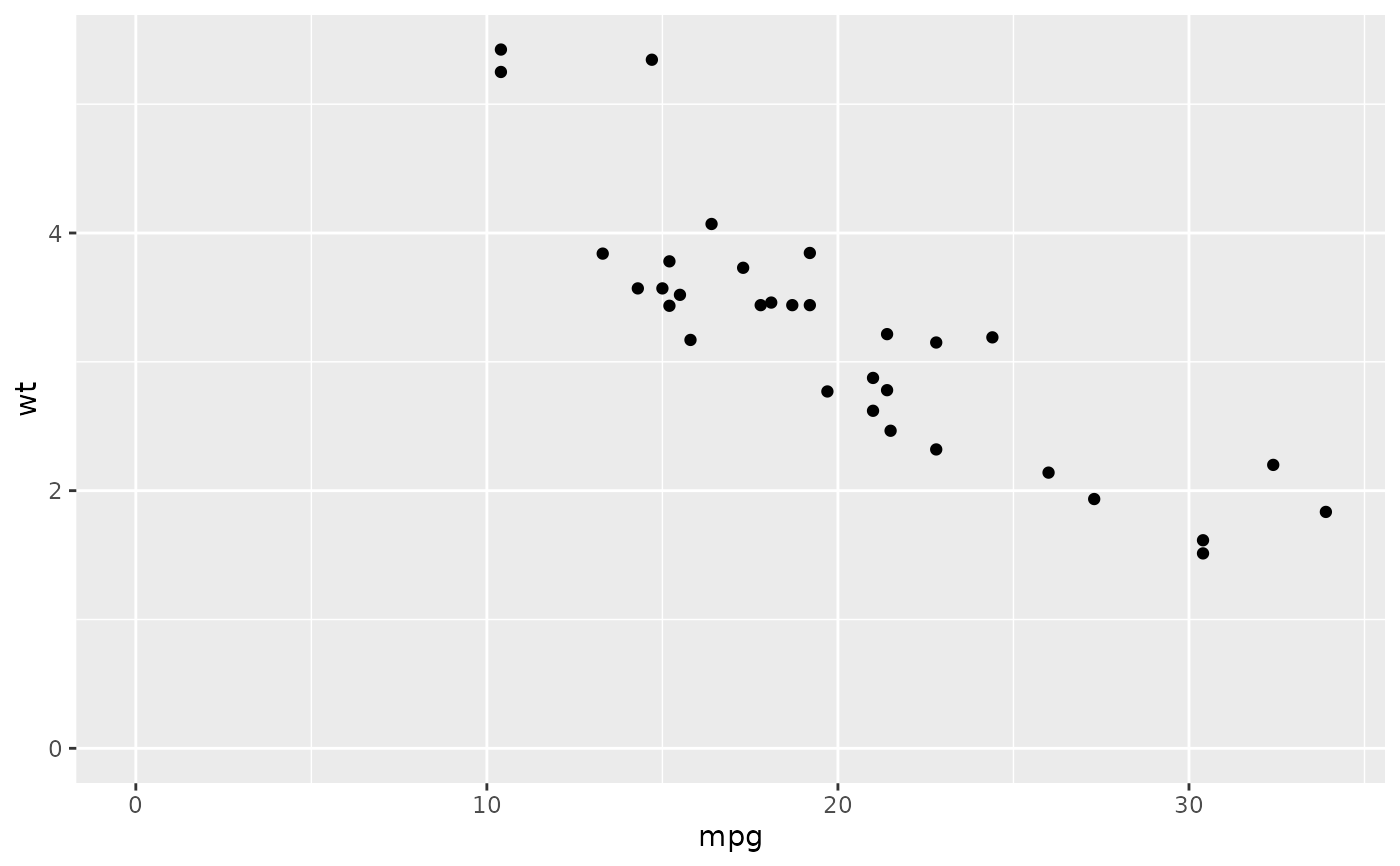ggplot(mtcars, aes(mpg, wt)) +
geom_point(aes(colour = cyl)) +
expand_limits(colour = seq(2, 10, by = 2))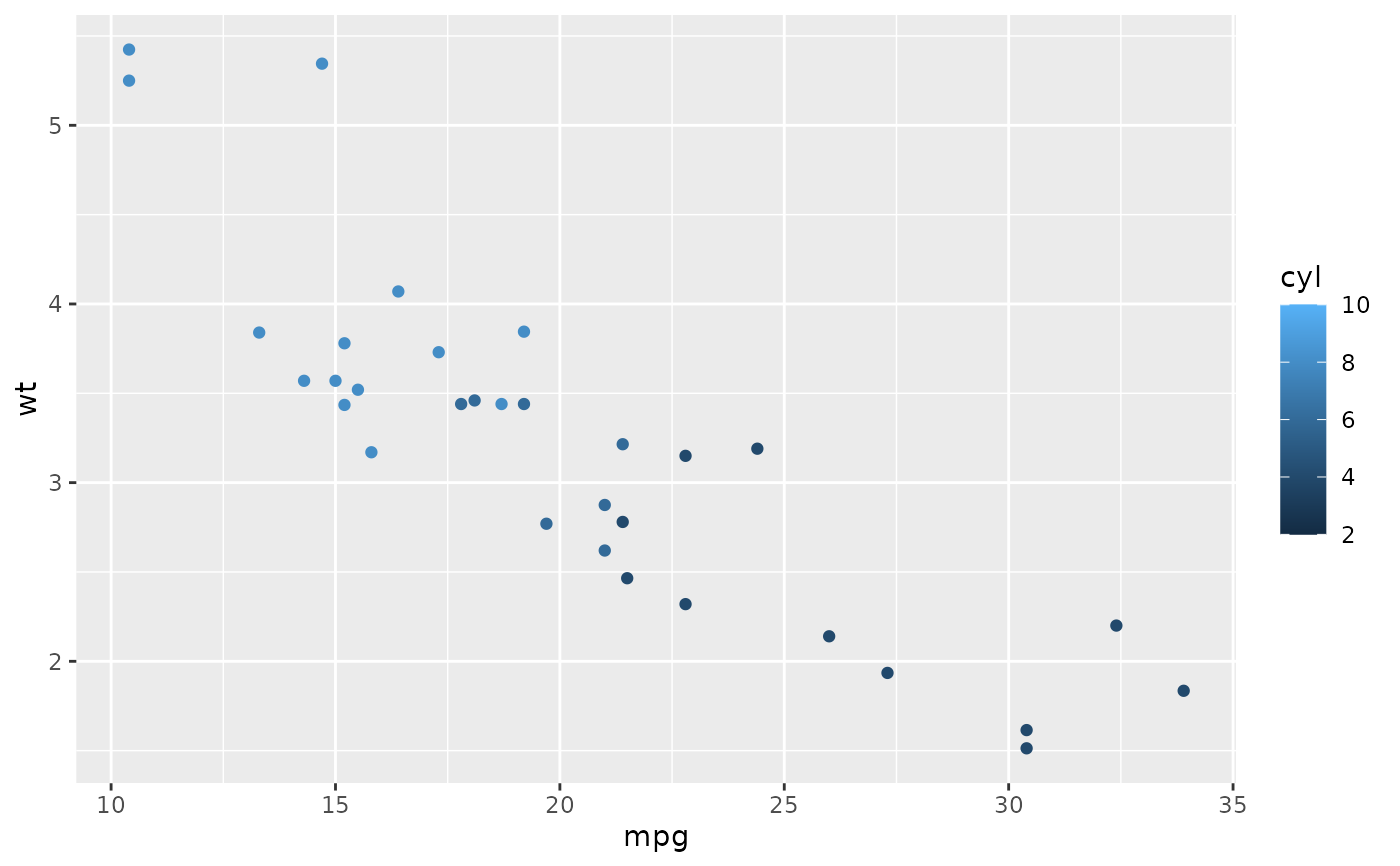ggplot(mtcars, aes(mpg, wt)) +
geom_point(aes(colour = factor(cyl))) +
expand_limits(colour = factor(seq(2, 10, by = 2)))